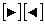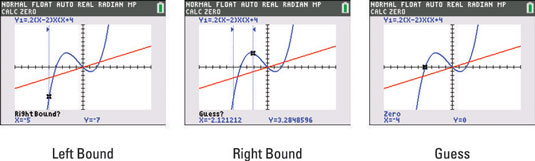##### Ti-84 Plus Graphing Calculator For Dummies, 2nd EditionYou can use your TI-84 Plus calculator to find the zeroes of a function. The zeros of the function y = f(x) are the solutions to the equation f(x) = 0. Because y = 0 at these solutions, these zeros (solutions) are really just the x-coordinates of the x-intercepts of the graph of y = f(x). (An x-intercept is a point where the graph crosses or touches the x-axis.)

To find a zero of a function, perform the following steps:

1. Graph the function in a viewing window that contains the zeros of the function.

To get a viewing window containing a zero of the function, that zero must be between Xmin and Xmax and the x-intercept at that zero must be visible on the graph.

2. Press [2nd][TRACE] to access the Calculate menu.

3. Press  to select the zero option.

4. If necessary, repeatedly press the up- and down-arrow keys until the appropriate function appears in the border at the top of the screen.

5. Set the Left Bound for the zero you desire to find.

To do so, use thekeys to place the cursor on the graph a little to the left of the zero, and then press [ENTER]. Alternatively, you can enter a number and press [ENTER] to establish the Left Bound.

On the TI-84 Plus C, a Left Bound vertical line appears on the screen (as illustrated by the dotted line with a small triangular indicator in the first screen).6. Set the Right Bound for the zero.

To do so, use thekeys to place the cursor on the graph a little to the right of the zero, and then press [ENTER]. Alternatively, you can enter a number and press e to establish the Right Bound.

On the TI-84 Plus C, a Right Bound dotted line with a small triangular indicator appears on the screen, as shown in the second screen.

7. Tell the calculator where you guess the zero is located.

This guess is necessary because the calculator uses a numerical routine for finding a zero. The routine is an iterative process that requires a seed (guess) to get it started. The closer the seed is to the zero, the faster the routine finds the zero. To do this, use thekeys to place the cursor on the graph as close to the zero as possible, and then press [ENTER]. The value of the zero appears in the border at the bottom of the screen, as shown in the third screen.

The calculator uses scientific notation to denote really large or small numbers. For example, –0.00000001 is displayed on the calculator as –1E–8, and 0.000000005 is displayed as 5E–8.